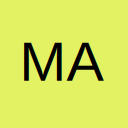# Option button = true then font colour to be changed

• Morning.
when I run the command button on my Userform I would like the font colour to be applied to the YES / NO text that will be entered on my worksheet depending on which option button was selected.

So on the Userform if option button 12 is selected I would like the text to be Red BUT if option button 13 is selected then the text should be Green.

• Sorry option button 1 and 2 not 12 and 13

• Hi

My mistake. Please see second post of mine.

• I understand that but i would like to use VBA

The code in place shown below would be the start of it but not sure how to write it

Code
1. If OptionButton1.Value = True Then .Cells(8, 10).Value = "YES": OptionButton1.Value = False
2. If OptionButton2.Value = True Then .Cells(8, 10).Value = "NO": OptionButton2.Value = False
• Try this

Code
1. If OptionButton1.Value = True Then
2. .Cells(8, 10).Value = "YES"
3. .Cells(8, 10).Interior.ColorIndex = vbRed
4. OptionButton1.Value = False
5. ElseIf OptionButton2.Value = True Then
6. .Cells(8, 10).Value = "NO"
7. .Cells(8, 10).Interior.ColorIndex = vbGreen
8. OptionButton2.Value = False
9. End If
• Hi,

Do i replace this.

Code
1. If OptionButton1.Value = True Then .Cells(8, 10).Value = "YES": OptionButton1.Value = False
2. If OptionButton2.Value = True Then .Cells(8, 10).Value = "NO": OptionButton2.Value = False

With this.

Code
1. If OptionButton1.Value = True Then
2. .Cells(8, 10).Value = "YES"
3. .Cells(8, 10).Interior.ColorIndex = vbRed
4. OptionButton1.Value = False
5. ElseIf OptionButton2.Value = True Then
6. .Cells(8, 10).Value = "NO"
7. .Cells(8, 10).Interior.ColorIndex = vbGreen
8. OptionButton2.Value = False
9. End If

I ask because i then get subscript of range